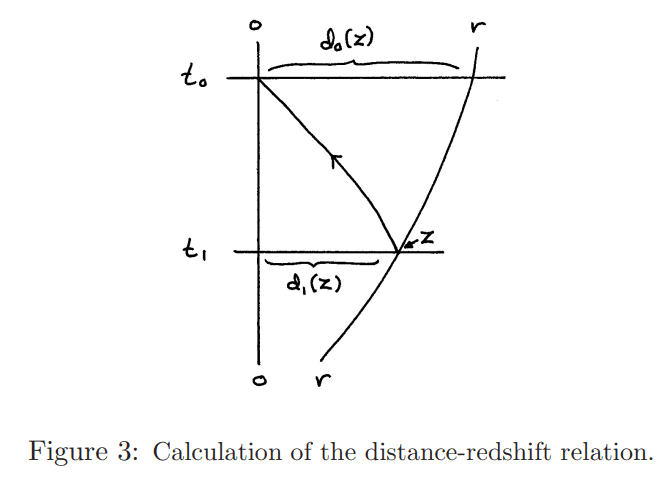# Understanding distances in Cosmology

• A
• Arman777

#### Arman777

Gold MemberI am trying to understand this graph but I am confused about the distance definitions. So there's an object located at a comoving distance ##r##.

The proper distance of the object at ##z'## can be written as
$$d(z{'}) = \frac{1}{1+z{'}}\int_0^{z{'}} \frac{dz{'}}{H(z{'})}$$

In this case,

$$d_1(z) = \frac{1}{1+z}\int_0^z \frac{dz}{H(z)}$$

and

$$d_0(z) = \int_0^z \frac{dz}{H(z)}$$

And we can also define the conformal time where it represents the distance taken by photon from the distant galaxy to us and can be written as

$$\eta(z) = \int_0^z \frac{dz}{H(z)}$$

There is also lookback time/distance but I am not sure it's same as the conformal time or not...

Are these definition that I have made are correct ?

•